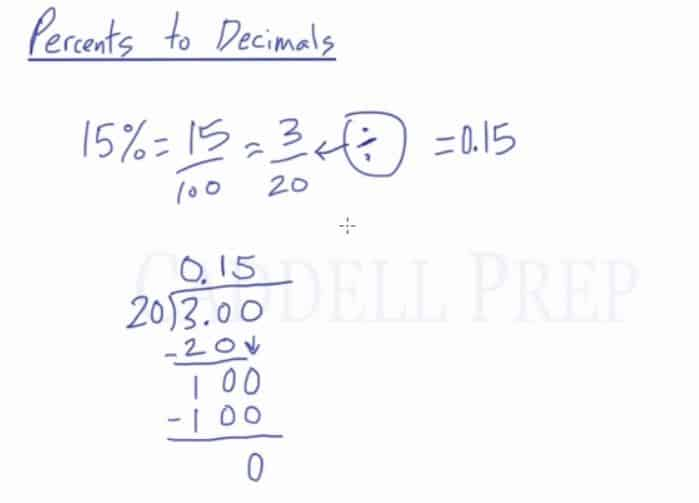## Examples of Representing a Percent as a Decimal

### Example 1$26\%$

We know that$26\% = \dfrac{26}{100}$

Then we simplify the fraction$\dfrac{26}{100}=\dfrac{13}{50}$

Since we want to convert it to decimals,$\dfrac{13}{50}= 13 \div 50$

Therefore,$13 \div 50$ is$0.26$

### Example 2$55\%$

We know that$55\% = \dfrac{55}{100}$

Then we simplify the fraction$\dfrac{55}{100}=\dfrac{11}{20}$

Since we want to convert it to decimals,$\dfrac{11}{20}= 11 \div 20$

Therefore,$11 \div 20$ is$0.55$

## Video-Lesson Transcript

Let us now discuss how to convert a percent into a decimal. Let’s write a percent as a decimal. After you finish this lesson, view all of our Pre-Algebra lessons and practice problems.

We now know that$15\% = \dfrac{15}{100}$ which we can further reduced to$= \dfrac{3}{20}$.

Since we want to convert it to decimals, please understand that$\dfrac{3}{20}= 3 \div 20$.

So if we divide 3 by 20 we will come up with the decimal equivalent of$15\%$.

So let’s do$3 \div20$ and the answer is$0.15$.

If you look at it,$15\%$ is the same as$0.15$.There’s a proper way to read$0.15$.

It is read as ‘fifteen hundredths’.

What do hundredths mean? This is the tenths spot and this is the hundredths spot.

Fifteen hundredths means$\dfrac{15}{100}$.

Right off the bat, we can write$15\% = \dfrac{15}{100} = 0.15$.

Another one is$3\% = \dfrac{3}{100} = 0.03$. It has to end in the hundredths spot. It is$\neq 0.3$.

So a quick way to convert percent to decimal is to move two decimal spaces from right to left.